### Solving Pell's equation without irrational numbers

The algorithm is due to Norman J. Wildberger at J. Integer Sequences, Vol. 13 (2010) 10.4.3. The BCMATH version is based on the BC version.

Here D > 1 is a nonsquare integer. We construct matrices Ak =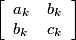with ak > 0 > ck with bk2 - akck = D and unimodular matrices Nk, k ≥ 0.
Starting with A0 =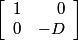and N0 = I0, and Nk = M1 ⋯ Mk if k ≥ 1, where Mi = L =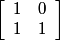or R =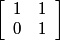and M1 = R, we choose Mk+1 = R if ak + 2bk + ck < 0, and choose Mk+1 = L if ak + 2bk + ck > 0.

We have NktA0Nk = Ak for k ≥ 0.

For some n > 0, NntA0Nn=A0, where Nn is a positive matrix of the form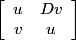and (u,v) is the least positive solution of Pell's equation. In the factorization of Nn, the Mi form a palindromic sequence.

The negative Pell equation will be soluble if and only if there exists a k such that Ak =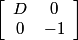, in which case, Ak will be at the centre of symmetry and Nk will have the form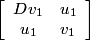. Then (u, v) = (u12 + Dv12, 2u1v1) and (u1, v1) will be the least positive solution of the negative Pell equation.

Note that in Wildberger's paper, on page 7, the equation N = MMt is not correct and should be replaced by N = MM+, where M+ =.

Wildberger does not prove that the solutions found are the least positive solutions, but this can be demonstrated by considering George Raney's L-R factorization algorithm applied to matrices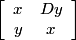and, where x2 - Dy2 = 1 and x12 - Dy12 = 1.

E = 0 prints the least positive solution of Pell's equation x2 - Dy2 = 1 and determines in the negative Pell equation x2 - Dy2 = -1 is soluble, in which case the least positive solution is found.

Enter d (1 < d < 1015 and non-square:)
Enter E (0 or 1):